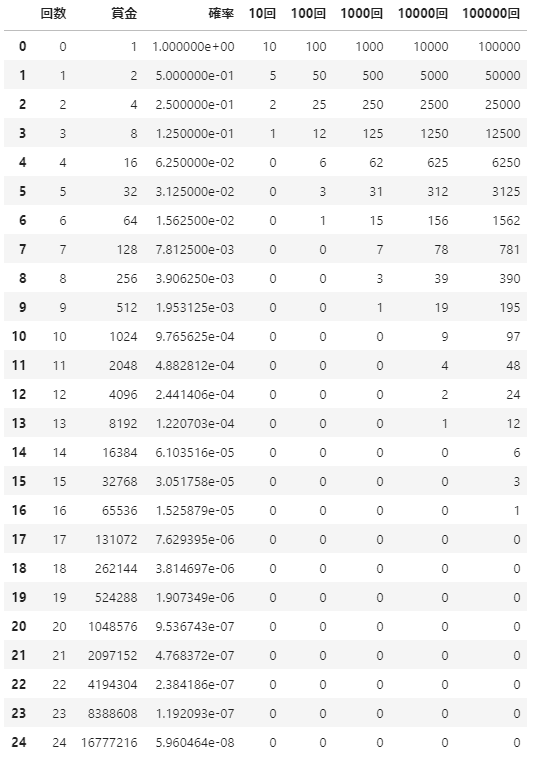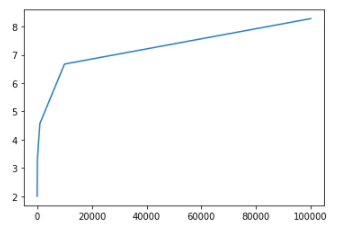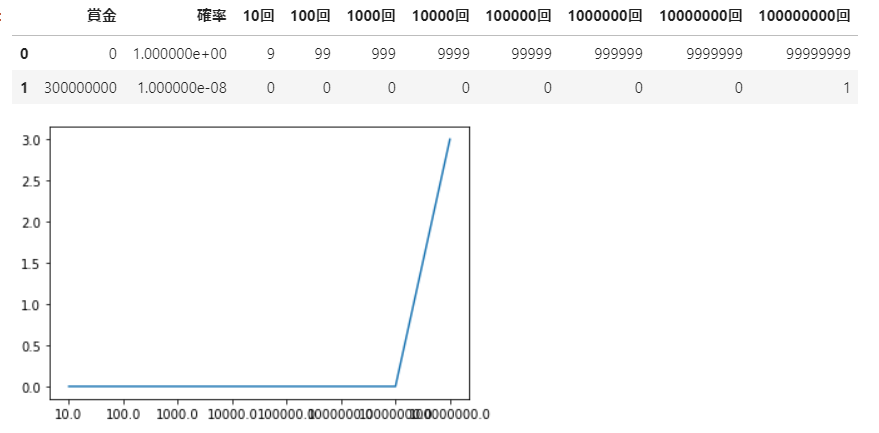In this post, I will write down my thoughts with reference to the wiki of the St. Petersburg paradox.

## Contents of the wiki

Suppose you have a game where you keep throwing unbiased coins [Note 1] until the front comes out and you get a prize when the front comes out. The prize money you can get is 1 yen when the front comes out the first time [Note 2], 2 yen when the back comes out the first time and doubles when the front comes out the second time, and the back comes out until the second time and the front is the first time It is a game in which you can get double the prize money, such as 4 yen, which is doubled when you get the prize, and 8 yen, which is doubled when the front is shown for the first time in the 4th time.

In other words, if the number of throws before the first appearance of the table is n, you will get 2n-1 yen. If the table appears for the first time in the 10th time, you will receive 512 yen, if the table appears for the first time in the 20th time, you will receive 524,288 yen, and if the table appears for the first time in the 30th time, you will receive 536,870,912 yen. Here, if a participation fee (= stake) is required for this game, how many yen can it be said that it is not a loss to pay the participation fee [Note 3]?

Mathematically, in this kind of problem, the expected value of the prize money is calculated, and if the participation fee is less than the expected value, it is judged that the participants will not lose. However, when we calculate the expected value of the prize money in this problem, the value diverges infinitely. That is, if the expected value is W

W=\sum _{k=1}^{\infty }\left({\frac {1}{2^{k}}}\cdot 2^{k-1}\right)={\frac {1}{2}}+{\frac {1}{2}}+{\frac {1}{2}}+{\frac {1}{2}}+\cdots =\infty

Will be. Therefore, judging from the expected value, it can be concluded that no matter how large the participation fee (= stake) is, you should participate. However, in reality, in this game, there is only a 1/2 chance of getting a prize of 1 yen, a 1/4 chance of getting 2 yen, and a 1/1024 chance of getting a prize of 512 yen (the prize is only 512 yen or less). The probability is 1023/1024). Therefore, it is intuitive that it cannot be so profitable. This is the reason why this problem is a paradox.

## Consideration

"Therefore, it is intuitively understood that it cannot be so profitable." The reason for judging that it is not profitable is due to the "utility" that will appear later, and if the utility is ignored, it will be a lot of money. However, it is better to participate. For example, if the participation fee is 500 million yen, it is an exceptional amount for a game if the utility is ignored. However, considering the utility, even if 500 million is a huge amount, in most cases Is a loss of 500 million and just doesn't want to participate. To put it more simply, we will first distribute 100 trillion yen (I'm glad I got it with 100% probability!). Next, 100 trillion lottery tickets are sold for 100 trillion yen, and only one lottery ticket is sold. There is a bet that the person who draws can get 2 x 100 trillion x 100 trillion yen (only one piece can be bought in possession and cannot be bought up). Should I do it because it doubles as the expected value? When you say that, you don't do it. "Therefore, you can intuitively understand that it cannot be so profitable." At this point, it feels intuitively regardless of whether the expected value is ∞. It is the same as this example.

## Contents of the wiki

In reality, there is an upper limit to prize money. For example, let's assume that the property of the body is 100 million yen. If the bottom comes out 27 times in a row, the prize money will exceed 100 million yen, so the game should be discontinued when the back comes out 26 times. Then, the expected value is

{\displaystyle {\frac {1}{2}}\cdot 1+{\frac {1}{2^{2}}}\cdot 2+\cdots +{\frac {1}{2^{26}}}\cdot 2^{25}+{\frac {1}{2^{26}}}\cdot 2^{26}=14}

It will be 14 yen. By performing the same calculation, it can be seen that the expected value is within the range of several tens of yen at most in the realistic range, no matter how rich the body is.

However, as a thought experiment, we can assume that the body has infinite ability to pay, and if we cannot answer the question of how much participation fee should be paid in that case, the problem is completely Not resolved.

## Consideration

Isn't this the answer? For example, let's say the property of the body is $2 ^ {999999998}$ Yen. Then, the expected value will be 500 million yen. So there is no doubt that 500 million yen is an equal stake. So will we take part in this bet here? It is No when it is said. The reason for No is the "utility" mentioned above. Furthermore, this cannot be recognized as an equal stake because our property is too scarce. So let's assume that our property is also $2 ^ {999999998}$ Yen. Then, since it is 500 million yen, you will be able to play with this body on an equal footing. Therefore, if the property of the body is set to ∞, it becomes the paradox of St. Petersburg, and we only need the property of ∞ to play on an equal footing. If you still want to make this bet at a reasonable amount other than ∞, you can quietly set an upper limit on your property and play with the expected value you get.

## Organize

The reason why 500 million yen seems intuitively unreasonable above is that our property is too small compared to the property of the body. From the perspective of the body, this 500 million yen is a Chilean price, so I don't feel anything when I lose it, and of course I don't feel anything when I receive it. To make this even more intuitive, let's assume that the body and our property are equal to 1 million yen, and scale the stake accordingly. In other words, the property is now $10 ^ 6/2 ^ {999999998}$ Yen, so you can start the stake at $5 × 10 ^ {14}/2 ^ {999999998}$. It's very sloppy and easy to see, is it about $5 × 10 ^ {-2999999992}$ yen? Now you know from our point of view that the stakes are priced like Chile.

I'm sorry

# Or later

I have already reached my conclusion, so I ignored the rest of the story.

# Sorry python element

Make a code like this without cutting corners

def test(N):
out = 0
for i in range(N):
n = 0
r = -1
while n < 0.5:
n = np.random.rand()
r += 1
out += 2 ** r
return out / N


I finally decided the number of people and the number of times and tried sampling, but it was useless. Well, if ∞ people do n times and find the expected value, any number of n will be ∞, so is it natural? If you limit the number of people and sample roughly, a curve-like thing will appear, but the lower limit just follows it, and the expected value is definitely ∞ regardless of the number of times.

## There was also such a thing

If you make a table like this

import pandas as pd

df = pd.DataFrame()
df['Number of times'] = np.arange(25)
df['Prize money'] = 2**df['Number of times']
df['probability'] = 1/2**df['Number of times']
df['10 times'] = (df['probability'] * 10).astype('i')
df['100 times'] = (df['probability'] * 100).astype('i')
df['1000 times'] = (df['probability'] * 1000).astype('i')
df['10000 times'] = (df['probability'] * 10000).astype('i')
df['100000 times'] = (df['probability'] * 100000).astype('i')
df


I can do something like thisActually, if you make a graph by saying that it will approach this depending on the number of trials or that it is crazy

res = []
N = [10 * 10**i for i in range(5)]
for n in N:
res.append(np.sum(df[str(n)+'Times'] * df['Prize money']) / np.sum(df[str(n)+'Times']))
plt.plot(N, res)The expected value (laughter) transitions like this, so it's like "This changes depending on the number of trials!" Doesn't it change? If this goes through, the lottery should be distributed for free. Because if you do the same, the expected value (laughter) will be 0 yen halfway. With this kind of feeling, why are there many people who are fooled by things that can be understood in common sense by thinking in the lottery, even though it is like a word trick ... For example, "If the number of trials increases, it will fail" or "Lottery If you buy a lot of them, you will be hit. " It will be easier to hit, but the expected value will not change ...

## I tried it with rattling

It is assumed that the probability of hitting is 1/100 million rattle, the prize money is 300 million yen, and the ball once issued is reused. Let's use the table below to find a reasonable entry fee w.

df = pd.DataFrame()
df['Prize money'] = [0, 300000000]
df['probability'] = [1 - 1/100000000, 1/100000000]
N = [10 * 10**i for i in range(8)]
res = []
for n in N:
df[str(n)+'Times'] = (df['probability'] * n).astype('i')
res.append(np.sum(df[str(n)+'Times'] * df['Prize money']) / np.sum(df[str(n)+'Times']))
plt.plot(res)
dfOne of the points to understand a reasonable rattle stake is the number of times the game is repeated. In a nutshell, if you decide in advance how many times you want to repeat the game, you can set a relatively fair stake. When repeated infinitely, the expected value W of rattle converges to 3 yen. However, if you make a table, you can see that this game is 0 yen up to 100 million times, but the expected value will be 3 yen from 100 million times. In other words, this game is a special good whose participation fee changes with a certain number of times as a threshold. Let's think concretely. Let's assume that the number of participation is 1000 times. That is, the retailer of this game sells 1000 games as one set. The price at this time will be free. On the other hand, game participation rights are better bought in bulk than in bulk, so consumers will want to buy in bulk. Then, it is meaningless for the dealer to set a threshold for the participation fee, which is disadvantageous for the dealer. In other words, the more you sell the set, the more you lose, and it is predicted that someday you will be hit by a rattle ... Well, if you think this is strange, the wiki should also be strange. Maybe something similar to this is happening. As I say many times, the property at the base cannot be less than the expected value when fixed as $2 ^ {999999998}$ yen.

# Conclusion

If there is no cap, then there is no cap on a reasonable stake, and I think it's strange because we don't have the ability to pay ∞. If you want to set a reasonable stake other than ∞, you have no choice but to set an upper limit. Furthermore, the property of the body is set at $2 ^ {999999998}$, which puts an upper limit on the participants, and the expected value at that time is 500 million yen. It can be judged as an unreasonable setting, and it is not necessary to consider the discussion in the latter half of the wiki. (There seems to be room to think about why such a misunderstanding is occurring)

# Supplement

I'll just deny what I'm writing on the wiki. (It may be correct in theory in the expression of the true author, but I feel that the content in the wiki alone is not even controversial.)

"Game retailers sell 1000 games as a set. The price at this time is about 9969 (≈ 1000 log2 1000) yen." This has an upper limit on the property of the body in particular. You should be assuming no. If you fix the property of the body to $2 ^ {999999998}$ yen, the expected value will be 500 million yen, and if you set this expected value as a reasonable stake, you should have set an upper limit to make the body advantageous, but the stake is The strange thing happens that it rises (the stake also gives the body an advantage). Well, in reality, nothing strange has happened, simply because the price setting of about 9969 (≈ 1000 log2 1000) yen is unreasonable. (I think that no one would deny that the property of this body is fixed at $2 ^ {999999998}$ yen and the upper limit is set to a reasonable stake, but if there is an opinion to deny this, it would be great. I'm interested)